# FIN Chapter 6_V1.ppt

30 de May de 2023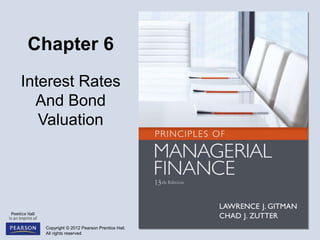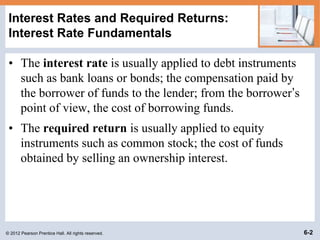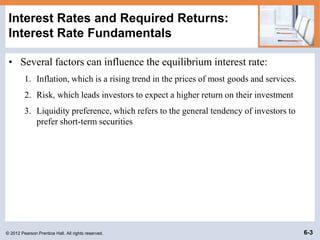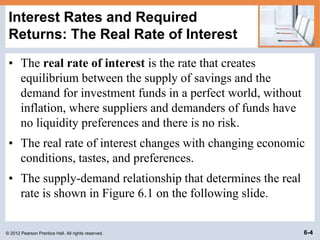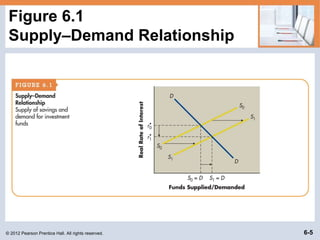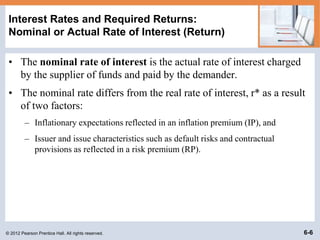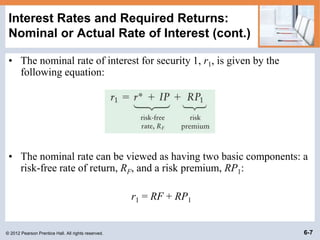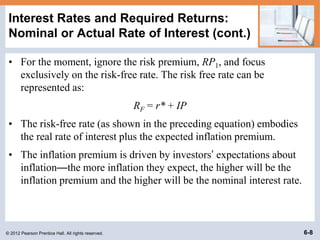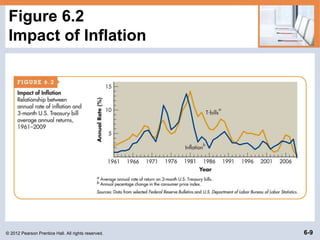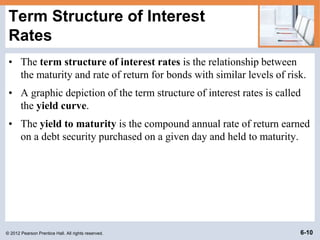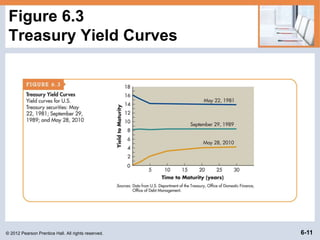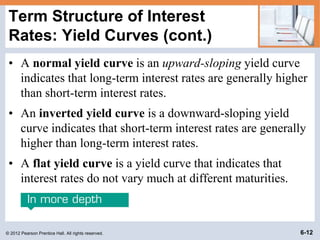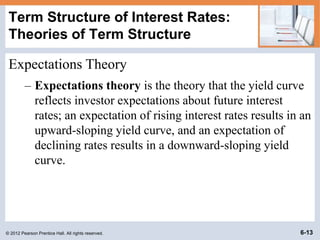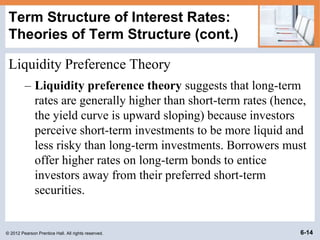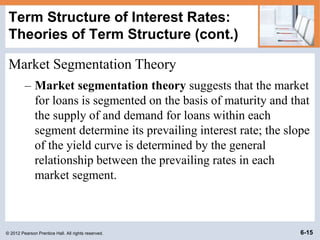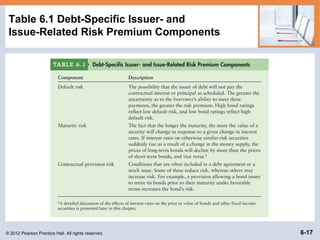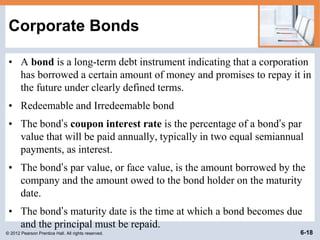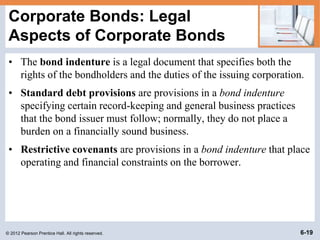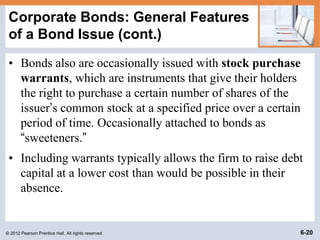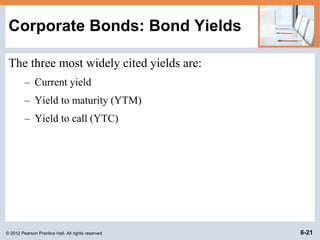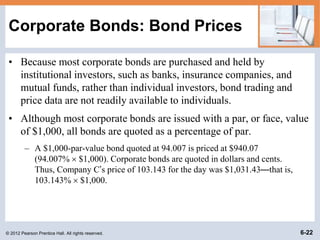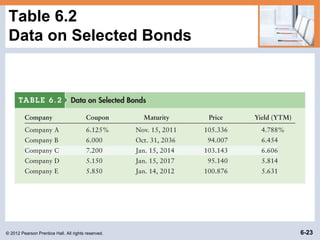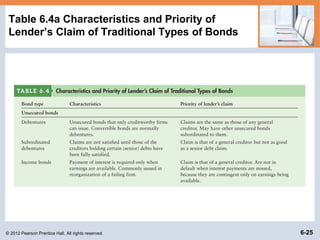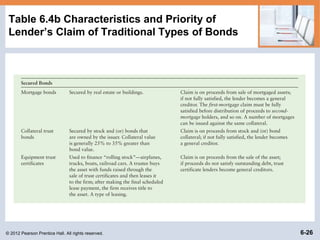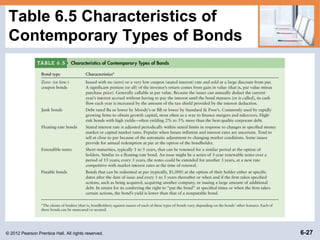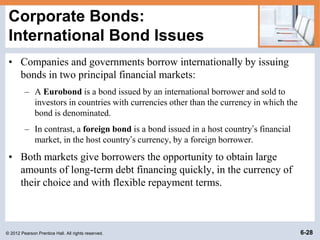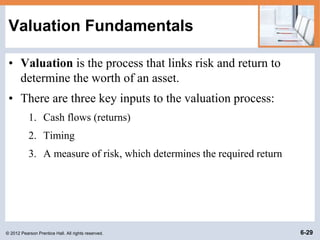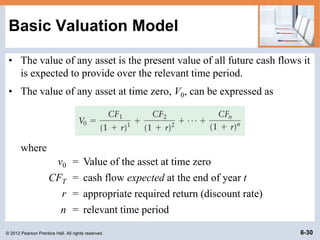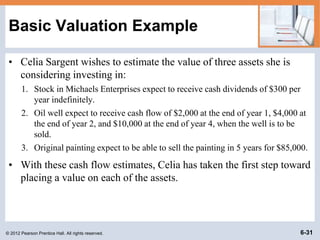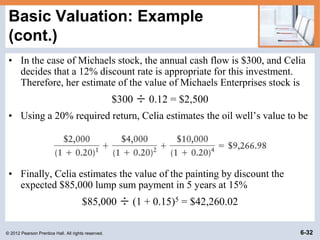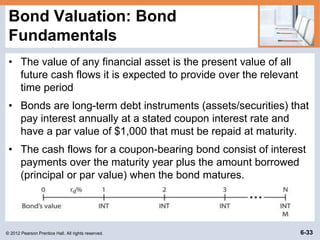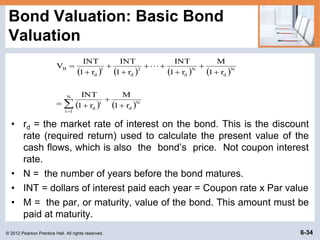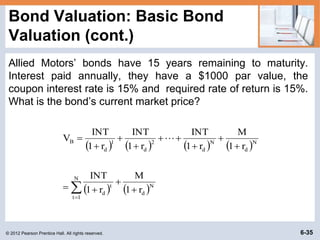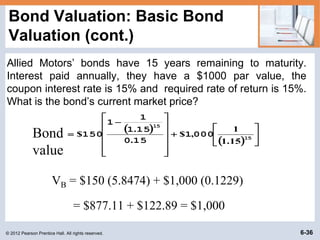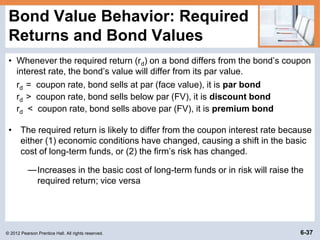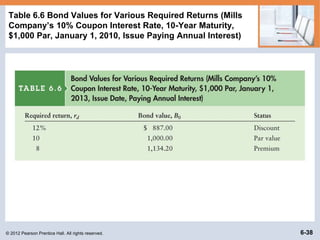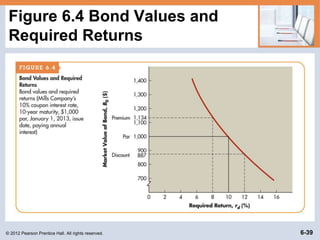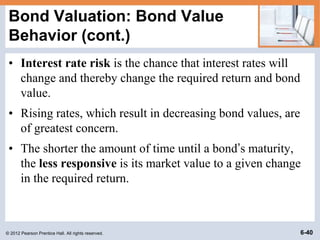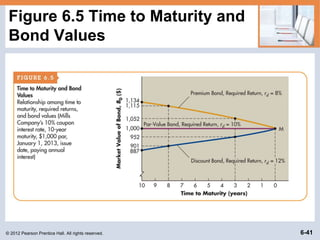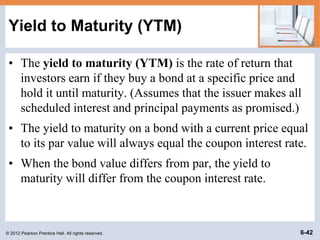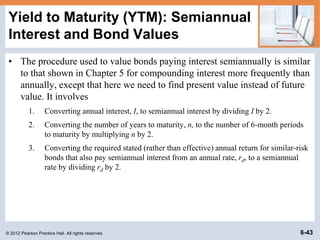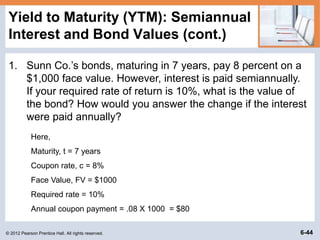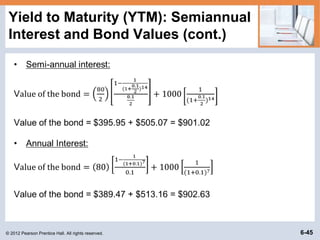1 de 45

### FIN Chapter 6_V1.ppt

• 2. © 2012 Pearson Prentice Hall. All rights reserved. 6-2 Interest Rates and Required Returns: Interest Rate Fundamentals • The interest rate is usually applied to debt instruments such as bank loans or bonds; the compensation paid by the borrower of funds to the lender; from the borrower’s point of view, the cost of borrowing funds. • The required return is usually applied to equity instruments such as common stock; the cost of funds obtained by selling an ownership interest.
• 3. © 2012 Pearson Prentice Hall. All rights reserved. 6-3 Interest Rates and Required Returns: Interest Rate Fundamentals • Several factors can influence the equilibrium interest rate: 1. Inflation, which is a rising trend in the prices of most goods and services. 2. Risk, which leads investors to expect a higher return on their investment 3. Liquidity preference, which refers to the general tendency of investors to prefer short-term securities
• 4. © 2012 Pearson Prentice Hall. All rights reserved. 6-4 Interest Rates and Required Returns: The Real Rate of Interest • The real rate of interest is the rate that creates equilibrium between the supply of savings and the demand for investment funds in a perfect world, without inflation, where suppliers and demanders of funds have no liquidity preferences and there is no risk. • The real rate of interest changes with changing economic conditions, tastes, and preferences. • The supply-demand relationship that determines the real rate is shown in Figure 6.1 on the following slide.
• 6. © 2012 Pearson Prentice Hall. All rights reserved. 6-6 Interest Rates and Required Returns: Nominal or Actual Rate of Interest (Return) • The nominal rate of interest is the actual rate of interest charged by the supplier of funds and paid by the demander. • The nominal rate differs from the real rate of interest, r* as a result of two factors: – Inflationary expectations reflected in an inflation premium (IP), and – Issuer and issue characteristics such as default risks and contractual provisions as reflected in a risk premium (RP).
• 7. © 2012 Pearson Prentice Hall. All rights reserved. 6-7 Interest Rates and Required Returns: Nominal or Actual Rate of Interest (cont.) • The nominal rate of interest for security 1, r1, is given by the following equation: • The nominal rate can be viewed as having two basic components: a risk-free rate of return, RF, and a risk premium, RP1: r1 = RF + RP1
• 8. © 2012 Pearson Prentice Hall. All rights reserved. 6-8 Interest Rates and Required Returns: Nominal or Actual Rate of Interest (cont.) • For the moment, ignore the risk premium, RP1, and focus exclusively on the risk-free rate. The risk free rate can be represented as: RF = r* + IP • The risk-free rate (as shown in the preceding equation) embodies the real rate of interest plus the expected inflation premium. • The inflation premium is driven by investors’ expectations about inflation—the more inflation they expect, the higher will be the inflation premium and the higher will be the nominal interest rate.
• 10. © 2012 Pearson Prentice Hall. All rights reserved. 6-10 Term Structure of Interest Rates • The term structure of interest rates is the relationship between the maturity and rate of return for bonds with similar levels of risk. • A graphic depiction of the term structure of interest rates is called the yield curve. • The yield to maturity is the compound annual rate of return earned on a debt security purchased on a given day and held to maturity.
• 12. © 2012 Pearson Prentice Hall. All rights reserved. 6-12 Term Structure of Interest Rates: Yield Curves (cont.) • A normal yield curve is an upward-sloping yield curve indicates that long-term interest rates are generally higher than short-term interest rates. • An inverted yield curve is a downward-sloping yield curve indicates that short-term interest rates are generally higher than long-term interest rates. • A flat yield curve is a yield curve that indicates that interest rates do not vary much at different maturities.
• 13. © 2012 Pearson Prentice Hall. All rights reserved. 6-13 Term Structure of Interest Rates: Theories of Term Structure Expectations Theory – Expectations theory is the theory that the yield curve reflects investor expectations about future interest rates; an expectation of rising interest rates results in an upward-sloping yield curve, and an expectation of declining rates results in a downward-sloping yield curve.
• 14. © 2012 Pearson Prentice Hall. All rights reserved. 6-14 Term Structure of Interest Rates: Theories of Term Structure (cont.) Liquidity Preference Theory – Liquidity preference theory suggests that long-term rates are generally higher than short-term rates (hence, the yield curve is upward sloping) because investors perceive short-term investments to be more liquid and less risky than long-term investments. Borrowers must offer higher rates on long-term bonds to entice investors away from their preferred short-term securities.
• 15. © 2012 Pearson Prentice Hall. All rights reserved. 6-15 Term Structure of Interest Rates: Theories of Term Structure (cont.) Market Segmentation Theory – Market segmentation theory suggests that the market for loans is segmented on the basis of maturity and that the supply of and demand for loans within each segment determine its prevailing interest rate; the slope of the yield curve is determined by the general relationship between the prevailing rates in each market segment.
• 18. © 2012 Pearson Prentice Hall. All rights reserved. 6-18 Corporate Bonds • A bond is a long-term debt instrument indicating that a corporation has borrowed a certain amount of money and promises to repay it in the future under clearly defined terms. • Redeemable and Irredeemable bond • The bond’s coupon interest rate is the percentage of a bond’s par value that will be paid annually, typically in two equal semiannual payments, as interest. • The bond’s par value, or face value, is the amount borrowed by the company and the amount owed to the bond holder on the maturity date. • The bond’s maturity date is the time at which a bond becomes due and the principal must be repaid.
• 19. © 2012 Pearson Prentice Hall. All rights reserved. 6-19 Corporate Bonds: Legal Aspects of Corporate Bonds • The bond indenture is a legal document that specifies both the rights of the bondholders and the duties of the issuing corporation. • Standard debt provisions are provisions in a bond indenture specifying certain record-keeping and general business practices that the bond issuer must follow; normally, they do not place a burden on a financially sound business. • Restrictive covenants are provisions in a bond indenture that place operating and financial constraints on the borrower.
• 20. © 2012 Pearson Prentice Hall. All rights reserved. 6-20 Corporate Bonds: General Features of a Bond Issue (cont.) • Bonds also are occasionally issued with stock purchase warrants, which are instruments that give their holders the right to purchase a certain number of shares of the issuer’s common stock at a specified price over a certain period of time. Occasionally attached to bonds as “sweeteners.” • Including warrants typically allows the firm to raise debt capital at a lower cost than would be possible in their absence.
• 21. © 2012 Pearson Prentice Hall. All rights reserved. 6-21 Corporate Bonds: Bond Yields The three most widely cited yields are: – Current yield – Yield to maturity (YTM) – Yield to call (YTC)
• 22. © 2012 Pearson Prentice Hall. All rights reserved. 6-22 Corporate Bonds: Bond Prices • Because most corporate bonds are purchased and held by institutional investors, such as banks, insurance companies, and mutual funds, rather than individual investors, bond trading and price data are not readily available to individuals. • Although most corporate bonds are issued with a par, or face, value of \$1,000, all bonds are quoted as a percentage of par. – A \$1,000-par-value bond quoted at 94.007 is priced at \$940.07 (94.007%  \$1,000). Corporate bonds are quoted in dollars and cents. Thus, Company C’s price of 103.143 for the day was \$1,031.43—that is, 103.143%  \$1,000.
• 28. © 2012 Pearson Prentice Hall. All rights reserved. 6-28 Corporate Bonds: International Bond Issues • Companies and governments borrow internationally by issuing bonds in two principal financial markets: – A Eurobond is a bond issued by an international borrower and sold to investors in countries with currencies other than the currency in which the bond is denominated. – In contrast, a foreign bond is a bond issued in a host country’s financial market, in the host country’s currency, by a foreign borrower. • Both markets give borrowers the opportunity to obtain large amounts of long-term debt financing quickly, in the currency of their choice and with flexible repayment terms.
• 29. © 2012 Pearson Prentice Hall. All rights reserved. 6-29 Valuation Fundamentals • Valuation is the process that links risk and return to determine the worth of an asset. • There are three key inputs to the valuation process: 1. Cash flows (returns) 2. Timing 3. A measure of risk, which determines the required return
• 30. © 2012 Pearson Prentice Hall. All rights reserved. 6-30 Basic Valuation Model • The value of any asset is the present value of all future cash flows it is expected to provide over the relevant time period. • The value of any asset at time zero, V0, can be expressed as where v0 = Value of the asset at time zero CFT = cash flow expected at the end of year t r = appropriate required return (discount rate) n = relevant time period
• 31. © 2012 Pearson Prentice Hall. All rights reserved. 6-31 Basic Valuation Example • Celia Sargent wishes to estimate the value of three assets she is considering investing in: 1. Stock in Michaels Enterprises expect to receive cash dividends of \$300 per year indefinitely. 2. Oil well expect to receive cash flow of \$2,000 at the end of year 1, \$4,000 at the end of year 2, and \$10,000 at the end of year 4, when the well is to be sold. 3. Original painting expect to be able to sell the painting in 5 years for \$85,000. • With these cash flow estimates, Celia has taken the first step toward placing a value on each of the assets.
• 32. © 2012 Pearson Prentice Hall. All rights reserved. 6-32 Basic Valuation: Example (cont.) • In the case of Michaels stock, the annual cash flow is \$300, and Celia decides that a 12% discount rate is appropriate for this investment. Therefore, her estimate of the value of Michaels Enterprises stock is \$300 ÷ 0.12 = \$2,500 • Using a 20% required return, Celia estimates the oil well’s value to be • Finally, Celia estimates the value of the painting by discount the expected \$85,000 lump sum payment in 5 years at 15% \$85,000 ÷ (1 + 0.15)5 = \$42,260.02
• 33. © 2012 Pearson Prentice Hall. All rights reserved. 6-33 Bond Valuation: Bond Fundamentals • The value of any financial asset is the present value of all future cash flows it is expected to provide over the relevant time period • Bonds are long-term debt instruments (assets/securities) that pay interest annually at a stated coupon interest rate and have a par value of \$1,000 that must be repaid at maturity. • The cash flows for a coupon-bearing bond consist of interest payments over the maturity year plus the amount borrowed (principal or par value) when the bond matures.
• 34.            N d N 1 t t d N d N d 2 d 1 d B r 1 M r 1 INT r 1 M r 1 INT r 1 INT r 1 INT V                 © 2012 Pearson Prentice Hall. All rights reserved. 6-34 Bond Valuation: Basic Bond Valuation • rd = the market rate of interest on the bond. This is the discount rate (required return) used to calculate the present value of the cash flows, which is also the bond’s price. Not coupon interest rate. • N = the number of years before the bond matures. • INT = dollars of interest paid each year = Coupon rate x Par value • M = the par, or maturity, value of the bond. This amount must be paid at maturity.
• 35. © 2012 Pearson Prentice Hall. All rights reserved. 6-35 Bond Valuation: Basic Bond Valuation (cont.) Allied Motors’ bonds have 15 years remaining to maturity. Interest paid annually, they have a \$1000 par value, the coupon interest rate is 15% and required rate of return is 15%. What is the bond’s current market price?            N d N 1 t t d N d N d 2 d 1 d B r 1 M r 1 INT r 1 M r 1 INT r 1 INT r 1 INT V                
• 36. © 2012 Pearson Prentice Hall. All rights reserved. 6-36 Bond Valuation: Basic Bond Valuation (cont.) Allied Motors’ bonds have 15 years remaining to maturity. Interest paid annually, they have a \$1000 par value, the coupon interest rate is 15% and required rate of return is 15%. What is the bond’s current market price?                          15 15 000 1 15 0 15 1 1 1 150 1.15 1 , \$ . . \$ Bond value VB = \$150 (5.8474) + \$1,000 (0.1229) = \$877.11 + \$122.89 = \$1,000
• 37. © 2012 Pearson Prentice Hall. All rights reserved. 6-37 Bond Value Behavior: Required Returns and Bond Values • Whenever the required return (rd) on a bond differs from the bond’s coupon interest rate, the bond’s value will differ from its par value. rd = coupon rate, bond sells at par (face value), it is par bond rd > coupon rate, bond sells below par (FV), it is discount bond rd < coupon rate, bond sells above par (FV), it is premium bond • The required return is likely to differ from the coupon interest rate because either (1) economic conditions have changed, causing a shift in the basic cost of long-term funds, or (2) the firm’s risk has changed. ─Increases in the basic cost of long-term funds or in risk will raise the required return; vice versa
• 38. © 2012 Pearson Prentice Hall. All rights reserved. 6-38 Table 6.6 Bond Values for Various Required Returns (Mills Company’s 10% Coupon Interest Rate, 10-Year Maturity, \$1,000 Par, January 1, 2010, Issue Paying Annual Interest)